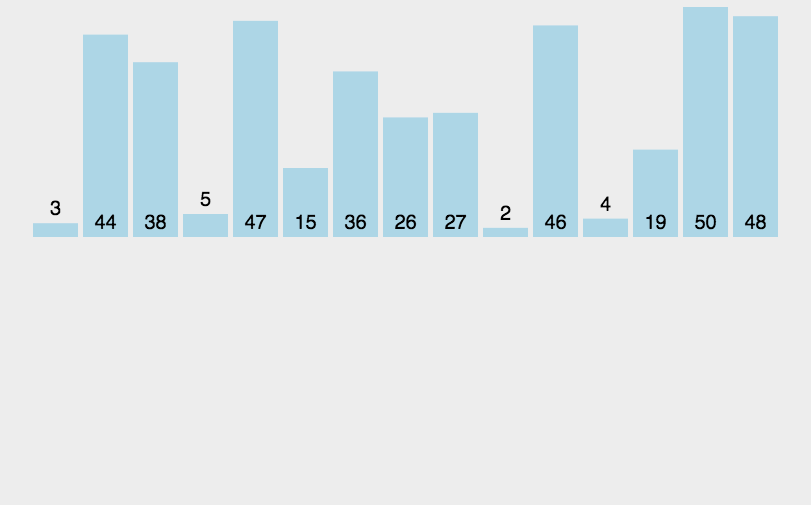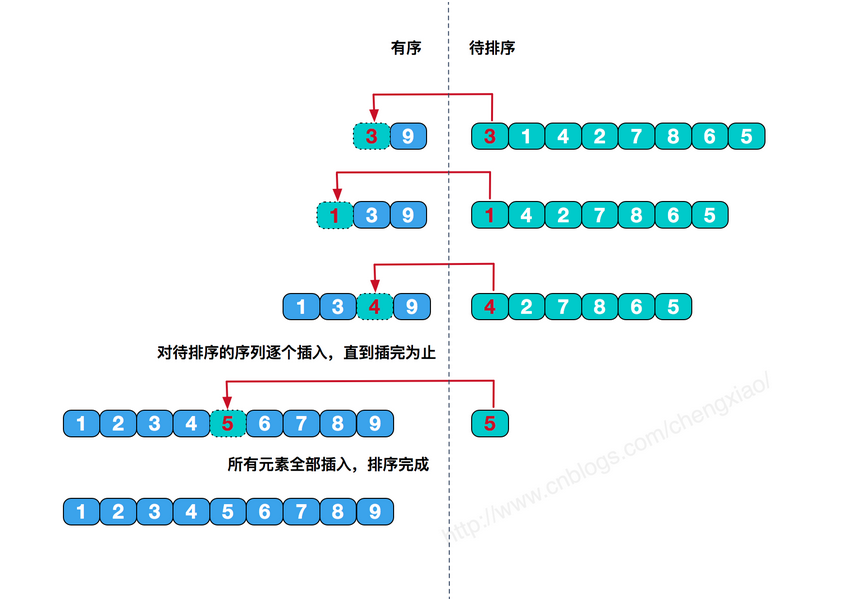## 插入法排序

※ 插入法排序原理※ 插入例項演算法分析
{3 -1 0 -8 2 1} 這是要進行插入排序的陣列

{3 -1 0 -8 2 1} -1 先把-1(陣列的第二個位置)拿出來當做要進行往左邊插入的值，

(左邊的值依次和這個值-1作比較得出)

{3 3 0 -8 2 1} -1 拿第一個位置的值3和-1比較，發現比-1大，那麼就把第一個位置

{-1 3 0 -8 2 1} -1 這個時候第一個位置

{-1 3 0 -8 2 1} 0 這次把0(陣列的第三個位置)拿出來當做要進行往左邊插入的值，

(左邊的值依次和這個值0作比較得出)

{-1 3 3 -8 2 1} 0 拿第二個位置的值3和0比較，發現比0大，那麼就把第二個位置

{-1 0 3 -8 2 1} 0 這個時候第二個位置

{-1 0 3 -8 2 1} -8 這次把-8(陣列的第四個位置)拿出來當做要進行往左邊插入的值，

(左邊的值依次和這個值-8作比較得出)

{-1 0 3 3 2 1} -8 拿第三個位置的值3和-8比較，發現比-8大，那麼就把第三個位置

{-1 0 0 3 2 1} -8 拿第二個位置的值0和-8比較，發現比-8大，那麼就把第二個位置

{-1 -1 0 3 2 1} -8 拿第一個位置的值-1和-8比較，發現比-8大，那麼就把第一個位置

{-8 -1 0 3 2 1} -8 這個時候第一個位置

{-8 -1 0 3 2 1} 2 這次把2(陣列的第五個位置)拿出來當做要進行往左邊插入的值，

(左邊的值依次和這個值2作比較得出)

{-8 -1 0 3 3 1} 2 拿第四個位置的值3和2比較，發現比2大，那麼就把第四個位置

{-8 -1 0 2 3 1} 2 這個時候第四個位置就有可能是我們要找的插入的位置,

{-8 -1 0 2 3 1} 1 這次把1(陣列的第六個位置)拿出來當做要進行往左邊插入的值，

(左邊的值依次和這個值1作比較得出)

{-8 -1 0 2 3 3} 1 拿第五個位置的值3和1比較，發現比1大，那麼就把第五個位置

{-8 -1 0 2 2 3} 1 拿第四個位置的值2和1比較，發現比1大，那麼就把第四個位置

{-8 -1 0 1 2 3} 1 這個時候第四個位置就有可能是我們要找的插入的位置,※ 實現程式碼如下：

``````package com.test.insertsort;
/*
* 插入排序演算法：
* 1、以陣列的某一位作為分隔位，比如index=1，假設左面的都是有序的.
*
* 2、將index位的資料拿出來，放到臨時變數裡，這時index位置就空出來了.
*
* 3、從leftindex=index-1開始將左面的資料與當前index位的資料（即temp）進行比較，如果array[leftindex]>temp,
* 則將array[leftindex]後移一位，即array[leftindex+1]=array[leftindex],此時leftindex就空出來了.
*
* 4、再用index-2(即leftindex=leftindex-1)位的資料和temp比，重複步驟3，
* 直到找到<=temp的資料或者比到了最左面（說明temp最小），停止比較，將temp放在當前空的位置上.
*
* 5、index向後挪1，即index=index+1，temp=array[index],重複步驟2-4，直到index=array.length,排序結束，
* 此時陣列中的資料即為從小到大的順序.
*
* @author bjh
*
*/
public class InsertSort {
private int[] array;
private int length;

public InsertSort(int[] array){
this.array = array;
this.length = array.length;
}

public void display(){
for(int a: array){
System.out.print(a+" ");
}
System.out.println();
}

/*
* 插入排序方法
*/
public void doInsertSort(){
for(int index = 1; index<length; index++){//外層向右的index，即作為比較物件的資料的index
int temp = array[index];//用作比較的資料
int leftindex = index-1;
while(leftindex>=0 && array[leftindex]>temp){//當比到最左邊或者遇到比temp小的資料時，結束迴圈
array[leftindex+1] = array[leftindex];
leftindex--;
}
array[leftindex+1] = temp;//把temp放到空位上
}
}

public static void main(String[] args){
int[] array = {38,65,97,76,13,27,49};
InsertSort is = new InsertSort(array);
System.out.println("排序前的資料為：");
is.display();
is.doInsertSort();
System.out.println("排序後的資料為：");
is.display();
}
}
``````
``````自己思考所寫：

package com.briup.Abstract;

import java.util.Arrays;

public class Test {
public static void main(String[] args) {
int[] array={12,73,45,69,35};
int i,j,temp;
for(i=1;i<array.length;i++) {
/*
* 第一個for迴圈
* 把陣列分成兩部分，右邊為未排序，左邊為已排序
* 記錄排序與未排序分割點temp（temp為下一個排序物件）
*/
temp=array[i];
for(j=i-1;j>=0;j--) {
/*
* 第二個for迴圈
* 將排序物件temp與已排序陣列比較
* 當temp比最近左邊的數大時（按從小到大循序排列時）
* 直接結束本次迴圈，進行下一個數排序
* 否則比左邊這個數小時將這個數後移，騰出這個數的位置
*/
if (temp > array[j]) {
break;
}else{
array[j+1] = array[j];
}
}
array[j+1]=temp;
}
System.out.println(Arrays.toString(array));
}
}

``````
``````三種排序彙總：

package com.briup.Abstract;

import java.util.Arrays;

public class A {
public int[] array;
public A(int[] array){
this.array=array;
}
//氣泡排序
public void test1() {
for(int i=1;i<array.length;i++) {
for(int j=0;j<array.length-i;j++) {
if(array[j]>array[j+1]) {
int temp=array[j];
array[j]=array[j+1];
array[j+1]=temp;
}
}
}
System.out.println(Arrays.toString(array));
}
//選擇排序
public void test2() {
int i,j,index;
for(i=0;i<array.length;i++) {
index=i;
for(j=i+1;j<array.length;j++) {
if(array[j]<array[index]) {
index=j;
}
}
int temp=array[i];
array[i]=array[index];
array[index]=temp;
}
System.out.println(Arrays.toString(array));
}
//插入排序
public void test3() {
int i,j,temp;
for(i=1;i<array.length;i++) {
temp=array[i];
for(j=i-1;j>=0;j--) {
if(temp>array[j]) {
break;
}else {
array[j+1]=array[j];
}
}
array[j+1]=temp;
}
System.out.println(Arrays.toString(array));
}
public static void main(String[] args) {
int[] array={23,34,25,66,21};
A a=new A(array);
a.test3();
}
}

``````